# 5.1 Introduction

The optimization field for TCAD applications  can be categorized into three major tasks. These are inverse modeling , calibration of simulator models [81,82] and the tuning of certain process parameters .

A TCAD optimization task is an iterative minimization or maximization process, where the optimizer controls a set of free parameters of a model within certain upper and lower bounds to minimize or maximize a given target. A simulator that takes the model as input is used to compute an error vector. The target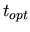is then computed as the quadratic mean of the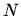dimensional error vector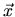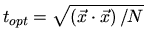(5.1)

The error vector is a modified relative error that is scaled to values between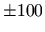to avoid numerical overflows. Depending on the optimization algorithm either the above given target or the error vector is passed to the optimizer which changes the free parameters according to its optimization algorithm in order to take a step towards the desired minimum or maximum.

Several iterations are performed until a truncation criterion is reached. For the case of a local optimizer the criterion is usually a minimum change of the target value. For a global optimizer additional criteria like, e.g. a maximum iteration number might be defined. An optimization framework integrates optimizer and simulator and spreads the simulation jobs over a cluster of workstations. Fig. 5.1 depicts this scenario. Several concrete sub-problems of this abstract optimization task exists which are briefly sketched in the following sections.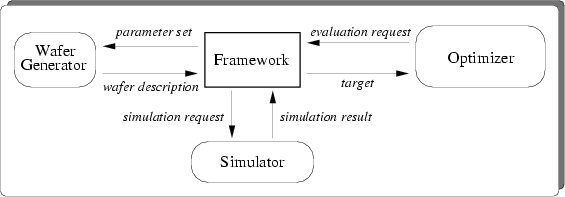Subsections

2003-03-27